Browse by Topic
Related Topics

# Graph Theory and Finance in Mathematica

Diversification is a way for investors to reduce investment risk. The asset values within a well-diversified portfolio do not move up and down in perfect synchrony. Instead, when some assets’ values move up, others tend to move down, evening out large, portfolio-wide fluctuations and thus reducing risk.

A simple way to explore diversification within the stock market is to invest in stocks from different sectors or different geographic regions. Beyond stocks, investors can consider diversification in different asset classes such as bonds, commodities, or real estate.

The following chart shows the S&P 500 and Dow Jones Industrials indices, indicators of return that move in sync with each other. You can download the Computable Document Format (CDF) version of this post below to execute this code yourself.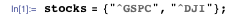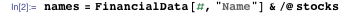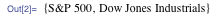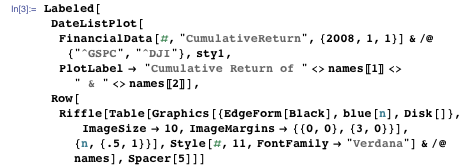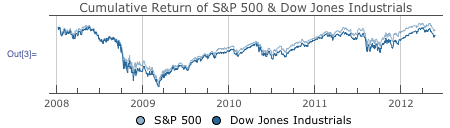Cumulative return shows how much an investment changes over time. The similarity of the two plots above shows the highly correlated nature of S&P 500 and Dow Jones Industrials.

The numerical correlation is nearly 1, an indication that their returns track each other extremely well.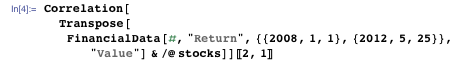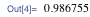Therefore, allocating an investment between Dow Jones Industrials and S&P 500 is not a good strategy for diversification.

In order to achieve effective diversification, we need to find asset classes with return correlations that are either small or negative, indicating that their returns either don’t track each other at all or move in opposite directions. Below, I have selected three asset classes—stock, commodity, and fixed income. Specifically, I’ve chosen the Dow Jones Industrials (DJI), gold (GLD), and the US Treasury Index Fund (TUZ) as a sample portfolio. Let’s calculate the correlations among their returns since 2010.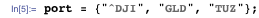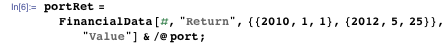The correlation matrix shows how pairs of assets are related: a value of 1 indicates that the corresponding pair of assets go up and down in perfect synchronization, a value of 0 indicates there is no relationship between their fluctuations, and a value of -1 indicates that when one goes up, the other goes down by the same amount.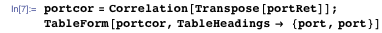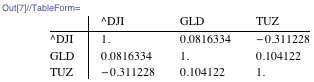Thus you can see that gold and the Dow Jones Industrials’ returns are hardly related at all, while the Treasury Fund Index tends to move in somewhat the opposite direction of the Dow Jones Industrials.

A matrix is helpful in that it provides precise information. However, once we expand our search to a wider investment universe, a large matrix will be cumbersome to interpret. Can we visually represent this correlation information? Graph theory provides a good solution.

From the Graphs and Networks: Concepts and Applications Wolfram Training course I attended recently, I learned that AdjacencyGraph gives a graph representation of a matrix. To illustrate, I first define matrix m: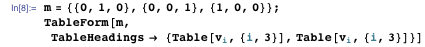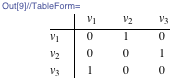In this matrix, 1 means a pair of vertices is connected, while 0 means that it is not. An arrow connects the two nodes of the corresponding graph exactly when there is a 1 in the corresponding location of the adjacency matrix. In matrix m above, vertex 1 (reading from the column of matrix) and vertex 2 (reading from the row of matrix) are connected. Correspondingly, an arrow is drawn from vertex 1 to vertex 2 in the adjacency graph. Stepping through the rows and columns of matrix m, the adjacency graph of matrix m is completed.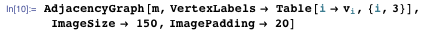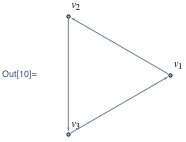To visualize relationships between returns in the portfolio above as a graph, I start with the correlation matrix. Since the correlation matrix is already in a matrix form, all I need to do is to turn it into something that can be represented by AdjacencyGraph. To do so, we can first define a threshold below which the entries in the correlation matrix will be 0 and above which the entries will be 1.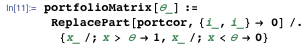Here I define the threshold to be 0.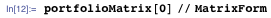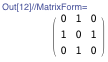From those thresholded correlations, AdjacencyGraph yields a graph.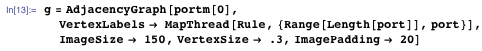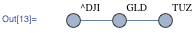This graph shows that the US Treasury Index Fund (TUZ) and the Dow Jones (DJI) are negatively correlated, since there is no edge connecting these two, and that the correlations among these two assets and gold are positive.

Let’s see if the graph theory technique can be applied to a bigger set of investment vehicles. I have first defined a bigger portfolio. It consists of all the members of the Dow Jones, a few commodities, and a few bonds. I am only interested in the correlation of returns since the beginning of this year.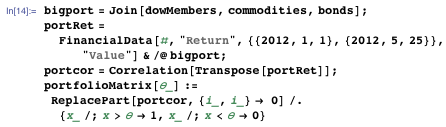In the graph below, I have chosen a correlation coefficient threshold of 0.58. If the return correlation between two assets is below 0.58, there is no edge connecting the assets. Thus, a pair of investments is connected if they are highly correlated.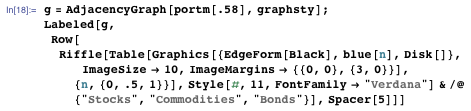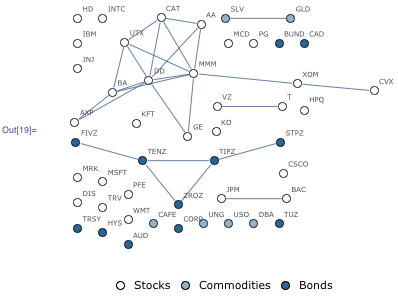As we can see, there are some distinct features of this graph. First of all, there are many assets whose returns are not strongly correlated with any other asset. They are the individual components within the graph. Secondly, there are five connected graphs. We can display those connected subgraphs for a closer inspection.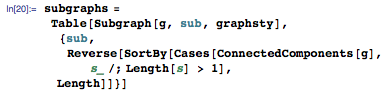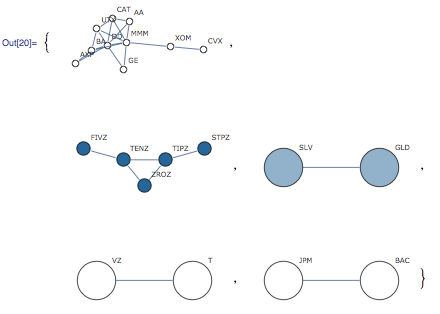The first subgraph consists of a few members of the Dow Jones Industrials.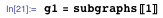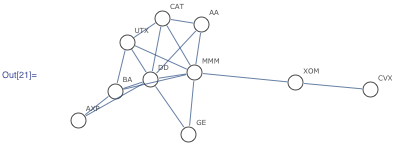The second subgraph consists of members of a few bond funds.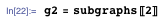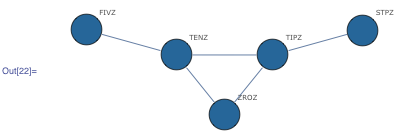The third and forth subgraphs are connected graphs with two vertices each. One of them connects silver and gold; the other connects Verizon and AT&T.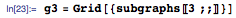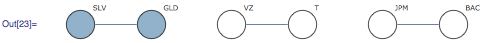These findings make sense. Traditionally, asset allocation between equity and bond provides a good diversification strategy. In the subgraphs, equities are indeed separated from bonds. What is interesting is that since the beginning of this year, Verizon/AT&T and J.P. Morgan Chase/Bank of America are in camps of their own, tracking each other closely, but unrelated to the rest of the Dow Jones Industrials members. Gold and silver are separated from the rest of the commodities.

One of the immediate analyses we can perform on the subgraph is to find out which groups of stocks tend to move in sync. Let’s take a look at g1, the connected subgraph that has a few members of the Dow Jones Industrials. To find subgroups of stocks in which every pair of stocks is connected, I can ask for the maximum clique within g1 using FindClique.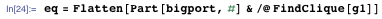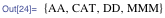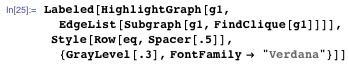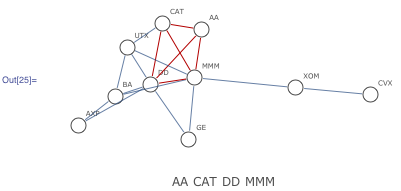The implication is that since the beginning of the year, those stocks have all tended to move in sync with each other. We can verify this claim from the cumulative return plots below.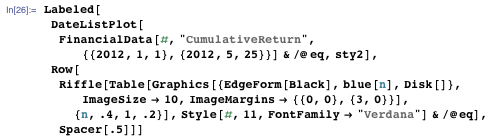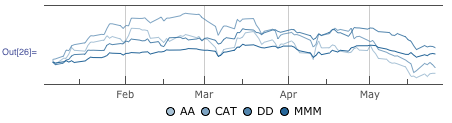However, for diversification purposes, we are looking for the assets whose returns are not highly correlated and therefore have correlation coefficients that are below the preset threshold value.

In a graph representation, there will be no connection between these diversified assets. Without a connection to other vertices, the diversified assets turn out to be the vertices that are never incident to the same edge.

There are many such independent sets. To include as many as assets as possible, we can use FindIndependentVertexSet, which finds a maximum number of independent vertices.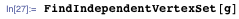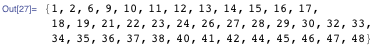Let’s highlight those vertices in our graph with stars: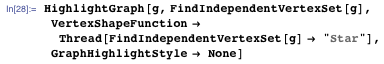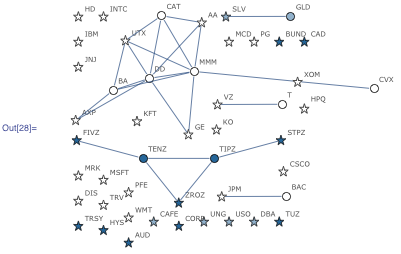If you were to choose a subset of investments from the stars in the graph, you’d have a well-diversified portfolio.

Graph theory has helped to determine which asset classes are highly correlated with one another and which are not. From the graph representation, the relationships between asset classes can be easily seen, the assets that are highly correlated with others can be quickly identified, and diversification between assets can be understood more intuitively.

We can certainly expand this type of analysis to include more asset classes and a longer (or different) time period. You can find all the necessary code in this post so you may explore more of the finance web.

A big thanks goes to our graph theory developer Charles Pooh for all the very helpful discussions on this blog post.